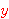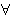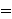New Foundations Explorer < Previous   Next > Nearby theorems Mirrors  >  Home  >  NFE Home  >  Th. List  >  ax9v Unicode version

Theorem ax9v 1655
 Description: Axiom B7 of [Tarski] p. 75, which requires thatandbe distinct. This trivial proof is intended merely to weaken axiom ax-9 1654 by adding a distinct variable restriction. From here on, ax-9 1654 should not be referenced directly by any other proof, so that theorem ax9 1949 will show that we can recover ax-9 1654 from this weaker version if it were an axiom (as it is in the case of Tarski). Note: Introducingas a distinct variable group "out of the blue" with no apparent justification has puzzled some people, but it is perfectly sound. All we are doing is adding an additional redundant requirement, no different from adding a redundant logical hypothesis, that results in a weakening of the theorem. This means that any future theorem that references ax9v 1655 must have a \$d specified for the two variables that get substituted forand. The \$d does not propagate "backwards" i.e. it does not impose a requirement on ax-9 1654. When possible, use of this theorem rather than ax9 1949 is preferred since its derivation from axioms is much shorter. (Contributed by NM, 7-Aug-2015.)
Assertion
Ref Expression
ax9vDistinct variable group:,Proof of Theorem ax9v
StepHypRef Expression
1 ax-9 1654 1Colors of variables: wff setvar class Syntax hints:wn 3wal 1540 This theorem was proved from axioms:  ax-9 1654 This theorem is referenced by:  a9ev  1656  spimw  1668  equidOLD  1677  sp  1747  spOLD  1748  spimehOLD  1821  equsalhwOLD  1839  cbv3hvOLD  1851  dvelimv  1939  ax10  1944  ax9  1949
 Copyright terms: Public domain W3C validator